# Entropy Generation Analysis of Carbon Nanotubes Nanofluid 3D Flow along a Nonlinear Inclined Stretching Sheet through Porous Media

Entropy Generation Analysis of Carbon Nanotubes Nanofluid 3D Flow along a Nonlinear Inclined Stretching Sheet through Porous Media

Shalini JainPreeti Gupta

Dept. of Mathemathics, University of Rajasthan, Jaipur-302004, Rajasthan, India

Dept. of Mathematics & Statistics, Manipal University Jaipur, Jaipur-303007, Rajasthan, India

Corresponding Author Email:
drshalinijainshah@gmail.com
Page:
131-138
|
DOI:
https://doi.org/10.18280/ijht.370116
21 February 2018
|
Accepted:
11 January 2019
|
Published:
31 March 2019
| Citation

OPEN ACCESS

Abstract:

Second law analysis for three-dimensional flow of water based CNTs nanofluid over an inclined stretching sheet subject to convective boundary condition in the presence of porous media has been done. For the analysis, we have taken two types of nanoparticles namely, single wall carbon nanotube (SWCNT) and multiwall carbon nanotube (MWCNT). A system of coupled non-linear differential equations for the flow is obtained by using similarity transformations through the conservation laws. Solution of resulting equations is obtained by Runge-Kutta fourth order mcethod with shooting technique. The effects of various physical parameters on flow and heat transfer characteristics as well as entropy generation has been investigated and displayed through graphs and tables.

Significance of the study:

The entropy generation analysis has been investigated in order to determine the optimal working condition for the given geometry under the considered boundary conditions. The mixture model with constant temperature properties was employed to simulate the nanofluid. Carbon nanotubes are hexagonally shaped arrangements of carbon atoms that have been rolled into tubes. Carbon nanotubes have high thermal conductivity, therefore, by adding nanoparticles or nanotubes in the base fluids the effective thermal conductivity of heat transfer fluids enhances.

Keywords:

CNTs, entropy, nonlinear inclined stretching sheet, porous media

1. Introduction

Nanofluids are used to increase the thermal conductivity and heat transfer rate of base fluids. The nanoparticles used in nanofluids are usually made of metals, carbides, oxides or carbon nanotubes. Carbon nanotubes are hexagonally shaped arrangements of carbon atoms that have been rolled into tubes. Carbon nanotubes were discovered in 1991 by Iijima. Carbon nanotubes have high thermal conductivity, exceptional mechanical strength and exceptional corrosion resistance. Their novel properties make them very useful in applications like microwave amplifier, nanotube sensors, nanotube transistors, hand held X-ray, field emission display, solar cell, lithium ion batteries and chemical sensors. Firstly nanofluids were introduced by Choi . He presented that solid nano size particles dropped in a carrier fluid to get a new type of complex fluid, known as nanofluid. Hayat et al.  studied the impact of margoni convection in viscous fluid flow of carbon water nanofluid. Aman et al.  investigated

four different types of molecular liquids are taken with CNTs Maxwell nanofluids in free convection flow. Hayat et al.  discussed three dimensional flow for homogenous-heterogeneous reactions for carbon nanotubes with porous media. Jain and Bohra  studied radiation and hall current effects on squeezing MHD nanofluid flow with a lower permeable stretching wall in a rotating channel.

A boundary-layer flow through a stretching surface has applicability in extrusion of plastic sheets, wire drawing, glass fiber production and paper production etc. Hayat et al.  studied three-dimensional stretching nonlinear surface magnetohydrodynamics nanofluid flow with a convective boundary condition. Mustafa et al.  discussed water based magnetite nanofluid rotating flow over a stretching surface by nonlinear thermal radiation. Gopal et al.  studied Joule’s and viscous dissipation on Casson fluid flow with inclined magnetic field over a chemical reacting stretching sheet. Kandasamy et al.  discussed MHD SWCNT type nanofluid flow considering both water and sea water as a base fluid.

A convective boundary condition increases the temperature and the thermal conductivity of nanofluids. Mahantesh et al.  studied radiative heat transfer in 3-D MHD nanofluid flow subject to the convective boundary condition over a stretching nonlinear sheet. Jain and Choudhary  studied the effect of MHD on boundary layer flow over exponentially shrinking sheet in the presence of porous media and slip. Chauhan and Olkha  studied heat transfer and slip flow of second-grade fluid through a porous medium past a stretching sheet with heat flux and power law surface temperature. Nayak  studied heat transfer effects of nanofluid flow by shrinking surface with convective condition. Jain and Bohra  studied Heat and mass transfer over a 3-D stretching inclined non-linear sheet with convective boundary conditions.

The entropy generation analysis has been investigated to determine the optimal working condition for the given geometry under the considered boundary conditions. The mixture model with constant temperature properties was employed to simulate the nanofluid. Results clearly showed that, the inclusion of nanoparticles produced a considerable increase of the heat transfer with respect to that of the base liquid. Heat transfer enhancement increased with the particle volume concentration. In thermal engineering, entropy generation minimization is applicable in devices such as air separators, chillers, fuel cell reactors, and thermal solar. Irreversibility phenomena associated with viscous dissipation, mass and heat transfer determined through entropy generation. Matin et al.  studied entropy in mixed convection MHD nanofluid flow over a sheet. Rehman et al.  discussed entropy of radioactive nanofluid flow with thermal slip. Das et al.  investigated entropy analysis of unsteady nanofluid flow past stretching sheet with convective condition. Chauhan and Kumar  studied Entropy generation impacts on non-Newtonian third grade fluid flow through partially filled annulus with temperature dependent viscosity and porous media. Shirley et al.  studied entropy analysis of nimonic 80A nanoparticles type nanofluid and stagnation point flow over a convectively stretching heated sheet. Vasanthakumari and Pondy  studied Mixed convection of titanium dioxide and silver nanofluids along inclined sheet with MHD and heat generation / suction effect.

The aim of this study is to examine the entropy generation for 3D flow of water based CNTs (SWCNTs and MWCNTs) nanofluid over an inclined stretching sheet subject to the convective boundary condition in the presence of porous media. The governing equations are solved using Runge-Kutta fourth order method with shooting technique. Effect of pertinent parameter has been obtained for velocity, temperature, and entropy generation profile. Results are discussed and displayed through graphs and tables.

2. Formulation of The Problem

Second law analysis over a inclined nonlinear stretching sheet with water based carbon nanotube nanofluid 3D flow through porous media under the convective boundary condition has been done. Velocity along x and y-directions are $U_w(x,y⁡)=c⁡(x+y)^n$, $V_w (x,y)=d(x+y)^n$. where n>0, c and d are positive constants. Schematic diagram shown in Figure 1.

## figure_1.png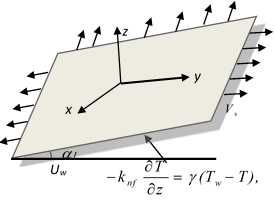Figure 1. Schematic diagram

The governing equations are:

$\frac{\partial u}{\partial x}+\frac{\partial v}{\partial y}+\frac{\partial w}{\partial z}=0$ (1)

$u\frac{\partial u}{\partial x}+v\frac{\partial u}{\partial y}+w\frac{\partial u}{\partial z}={{\upsilon }_{nf}}\frac{{{\partial }^{2}}u}{\partial {{z}^{2}}}-\frac{{{\upsilon }_{nf}}}{{{k}_{p}}}u+g\left[ {{\beta }_{T}}\left( T-{{T}_{\infty }} \right) \right]\cos \alpha$(2)

$u\frac{\partial v}{\partial x}+v\frac{\partial v}{\partial y}+w\frac{\partial v}{\partial z}={{\upsilon }_{nf}}\frac{{{\partial }^{2}}v}{\partial {{z}^{2}}}-\frac{{{\upsilon }_{nf}}}{{{k}_{p}}}v+g\left[ {{\beta }_{T}}\left( T-{{T}_{\infty }} \right) \right]sin\alpha$ (3)

$u\frac{\partial T}{\partial x}+v\frac{\partial T}{\partial y}+w\frac{\partial T}{\partial z}=\frac{{{k}_{nf}}}{{{\left( \rho {{c}_{p}} \right)}_{nf}}}\frac{{{\partial }^{2}}T}{\partial {{z}^{2}}}-\frac{1}{{{\left( \rho {{c}_{p}} \right)}_{nf}}}\frac{\partial {{q}_{r}}}{\partial Z}$  (4)

Here $u,v,w$  are fluid velocities along the directions $x,y,z$  respectively; $k_p$ is the permeability of porous medium; $β_T$ is the thermal expansion.

Under the boundary conditions are

at

$z=0,$, $u=c{{\left( x+y \right)}^{n}},$ $v=d{{\left( x+y \right)}^{n}},$ $w=0,$$-{{k}_{nf}}\frac{\partial T}{\partial z}=\gamma \left( {{T}_{w}}-T \right)$ (5)

at $z\to \infty$, $u\to 0$, $v\to 0$, $T\to {{T}_{∞}}$ (6)

where $μ_{nf},υ_{nf},ϕ,ρ_{nf},ρ_{CNT}$ is the viscosity of nanofluid, kinematic viscosity of nanofluid, volume fraction of nanoparticle, density of nanofluid, the density of carbon nanotube respectively. Thermo physical property of fluid and nano particles is given in Table 1.

${{\mu }_{nf}}=\frac{{{\mu }_{f}}}{{{\left( 1-\phi \right)}^{2.5}}},$${{\upsilon }_{nf}}=\frac{{{\mu }_{nf}}}{{{\rho }_{nf}}},$${{A}_{1}}=\frac{{{\rho }_{nf}}}{{{\rho }_{f}}}=\left( 1-\phi \right)+\phi \frac{{{\rho }_{CNT}}}{{{\rho }_{f}}}$,${{A}_{2}}=\frac{{{\left( \rho {{c}_{p}} \right)}_{nf}}}{{{\left( \rho {{c}_{p}} \right)}_{f}}}=\left( 1-\phi \right)+\phi \frac{{{\left( \rho {{c}_{p}} \right)}_{CNT}}}{{{\left( \rho {{c}_{p}} \right)}_{f}}}$ (7)

The effective thermal conductivity of nanofluid is expressed as

$\frac{{{k}_{nf}}}{{{k}_{f}}}=\left( \frac{1-\phi +2\phi \left( \frac{{{k}_{CNT}}}{{{k}_{CNT}}-{{k}_{f}}} \right)\ln \left( \frac{{{k}_{CNT}}+{{k}_{f}}}{2{{k}_{f}}} \right)}{1-\phi +2\phi \left( \frac{{{k}_{f}}}{{{k}_{CNT}}-{{k}_{f}}} \right)\ln \left( \frac{{{k}_{CNT}}+{{k}_{f}}}{2{{k}_{f}}} \right)} \right)$ (8)

Table 1. Thermophysical properties of base fluid and nanoparticles

 Thermophysical properties of base fluid and nanoparticles Physical characteristics Water (Base-fluid) SWCNT (nanoparticle) MWCNT (nanoparticle) ρ  (kg/m3) 997.1 2600 1600 $C_p$ (J/kgK) 4179 425 796 k (W/mK) 0.613 6600 3000

The Rosseland approximation is expressed as

${{q}_{r}}=-\frac{4{{\sigma }^{*}}}{3{{k}^{*}}}\frac{\partial {{T}^{4}}}{\partial z}\） (9) where σ* is the Stefen-Boltzmann constant and k* is the mean absorption coefficient. The temperature difference has been considered very small, so that T4 may be expressed as a linear function of temperature. \({{T}^{4}}\approx 4{{T}^{3}}_{\infty }T-3{{T}^{4}}_{\infty }$  (10)

Similarity transformations are

$u=c{{\left( x+y \right)}^{n}}f'(\eta ),$ $v=c{{\left( x+y \right)}^{n}}g'\left( \eta \right),$$\eta ={{\left( \frac{c\left( n+1 \right)}{2{{\upsilon }_{f}}} \right)}^{1/2}}{{\left( x+y \right)}^{\frac{n-1}{2}}}z,$$T-{{T}_{\infty }}=\left( {{T}_{w}}-{{T}_{\infty }} \right)\theta$

$w=-{{\left( \frac{c{{\upsilon }_{f}}\left( n+1 \right)}{2} \right)}^{1/2}}\left\{ \left( f+g \right)+\frac{n-1}{n+1}\eta \left( f'+g' \right) \right\}{{\left( x+y \right)}^{\frac{n-1}{2}}},$ (11)

On substituting equation (11) in Equation (1)–(4), equation (1) identically satisfies and equation (2)-(4) transformed into the following form:

\begin{align} & f'''+\left\{ \left( f+g \right)f''-\frac{2n}{n+1}\left( f'+g' \right)f'+\frac{2}{n+1}\delta \cos {{\alpha }_{1}}\theta \right\} \\ & {{(1-\phi )}^{2.5}}{{A}_{1}}-\frac{2K}{n+1}f'=0 \\ \end{align}  (12)

\begin{align} & g'''+\left\{ \left( f+g \right)g''-\frac{2n}{n+1}\left( f'+g' \right)g'+\frac{2}{n+1}\delta sin{{\alpha }_{1}}\theta \right\} \\ & {{(1-\phi )}^{2.5}}{{A}_{1}}-\frac{2K}{n+1}g'=0, \\ \end{align} (13)

$\frac{1}{{{A}_{2}}\Pr }\left( {{A}_{3}}+\frac{4}{3}R \right)\theta ''+\left( f+g \right)\theta '=0$ (14)

Under the boundary condition

at $η=0$, $f(0)=g(0)=0$, $f'(0)=1$, $g'(0)=α$, $\theta \left( 0 \right)=1+\frac{{{A}_{3}}}{{{B}_{i}}}\theta '\left( 0 \right)$

at η→∞, f'(∞)→0, g'(∞)→0, θ(∞)→0 (15)

where K is the local porosity parameter, α is the ratio parameter, R is the radiation parameter, $P_r$ is the Prandtl number. These non-dimensional variables are defined by

$K=\frac{{{\upsilon }_{f}}}{{{k}_{p}}c{{\left( x+y \right)}^{n-1}}}$, $\alpha =\frac{d}{c}$, $\Pr =\frac{{{\mu }_{f}}{{\left( {{c}_{p}} \right)}_{f}}}{{{k}_{f}}}$, $R=\frac{4{{\sigma }^{*}}{{T}_{\infty }}^{3}}{{{k}^{*}}{{k}_{f}}}$ (16)

3. Solution

The equations (12)-(14) under the boundary conditions (15) have been solved numerically by RK-4 method with shooting technique. Runge-Kutta fourth method need a finite domain 0≤η≤η, in this study we have chosen η=10 . The boundary value problems are changed into initial value problems, are defined as

f=f1, f'=f2, f''=f3, g=f4, g'⁡= f5, g''⁡= f6,

${{f}_{3}}'=-$ \left\{ \begin{align} & -\frac{2K}{n+1}{{f}_{2}}+{{(1-\phi)}^{2.5}}{{A}_{1}} \\ & ({{f}_{1}}+{{f}_{4}}){{f}_{3}}-\frac{2n}{n+1}({{f}_{2}}+{{f}_{5}}){{f}_{2}}+\frac{2}{n+1}\delta cos {{\alpha }_{1}}{{f}_{8}} \\ \end{align} \right\} (17)

${{f}_{6}}^{'}=-$ \left\{ \begin{align} & -\frac{2K}{n+1}{{f}_{5}}+{{(1-\phi)}^{2.5}}{{A}_{1}} \\ & ({{f}_{1}}+{{f}_{4}} ){{f}_{6}}-\frac{2n}{n+1}\left( {{f}_{2}}+{{f}_{5}} \right){{f}_{5}} \\ & +\frac{2}{n+1}\delta sin{{\alpha }_{1}}{{f}_{8}} \\ \end{align} \right\} (18)

${{f}_{8}}'$$=\frac{{{A}_{2}}\Pr }{( {{A}_{3}}+\frac{4}{3}R)}$$\{( {{f}_{1}}+{{f}_{4}} ){{f}_{_{8}}}\}$ (19)

Under the boundary condition

${{f}_{1}}\left( 0 \right)={{f}_{4}}\left( 0 \right)=0$, ${{f}_{2}}\left( 0 \right)=1,$ ${{f}_{5}}\left( 0 \right)=\alpha ,$ ${{f}_{7}}\left( 0 \right)=1+\frac{{{A}_{3}}}{{{B}_{i}}}{{f}_{8}}\left( 0 \right),$ ${{f}_{3}}(0)={{r}_{1}},{{f}_{6}}(0)={{r}_{2}}\,,{{f}_{8}}(0)={{r}_{3}},$ (20)

where, $r_1$, $r_2$ and $r_3$ are the initial guesses.

4. Entropy Generation

The local volumetric entropy equation rate of viscous incompressible fluid with porous medium is written as

\begin{align} & S''{{'}_{gen}}=\left\{ {{\left( \frac{\partial T}{\partial x} \right)}^{2}}+{{\left( \frac{\partial T}{\partial y} \right)}^{2}}+{{\left( \frac{\partial T}{\partial z} \right)}^{2}}+\frac{4}{3}R{{\left( \frac{\partial T}{\partial z} \right)}^{2}} \right\}\frac{{{k}_{nf}}}{{{T}_{\infty }}^{2}} \\ & +\frac{{{\mu }_{nf}}}{{{T}_{\infty }}}\left\{ {{\left( \frac{\partial u}{\partial z} \right)}^{2}}+{{\left( \frac{\partial v}{\partial z} \right)}^{2}} \right\}+\frac{1}{{{T}_{\infty }}}\frac{{{\mu }_{nf}}}{{{k}_{p}}}{{u}^{2}} \\ \end{align} (21)

The non-dimensional form of characteristic entropy generation rate is

$S''{{'}_{0}}=\frac{{{k}_{nf}}{{\left( {{T}_{w}}-{{T}_{\infty }} \right)}^{2}}}{{{T}_{\infty }}^{2}{{L}^{2}}}$  (22)

Hence the entropy generation number is

${{N}_{G}}=\frac{S''{{'}_{gen}}}{S''{{'}_{0}}}$ (23)

On using equations (21) and (22) in equation (23) reduces into the following equation

\begin{align} & {{N}_{G}}=\left\{ \frac{{{\left( n-1 \right)}^{2}}}{2}{{\eta }^{2}}+\left( \frac{n+1}{2} \right){{\operatorname{Re}}_{L}}\left( 1+\frac{4}{3}R \right) \right\}\theta {{'}^{2}} \\ & +\frac{{{\operatorname{Re}}_{L}}KBr}{{{\left( 1-\phi \right)}^{2.5}}\Omega {{A}_{3}}}f{{'}^{2}}+\left( \frac{n+1}{2} \right)\frac{Br{{\operatorname{Re}}_{L}}}{{{A}_{3}}{{\left( 1-\phi \right)}^{2.5}}\Omega }\left( f'{{'}^{2}}+g'{{'}^{2}} \right) \\ \end{align}(24)

where, $Re_L⁡$, $B_r$, Ω denotes the Reynolds number, Brinkman number, dimensionless temperature difference, respectively. These numbers can be expressed as

${{\operatorname{Re}}_{L}}=\frac{{{U}_{w}}L}{{{\upsilon }_{f}}}$, $Br=\frac{{{\mu }_{f}}{{U}_{w}}^{2}}{{{k}_{f}}\left( {{T}_{w}}-{{T}_{_{\infty }}} \right)}$, $\Omega =\frac{\left( {{T}_{w}}-{{T}_{\infty }} \right)}{{{T}_{\infty }}}$

5. Validation of The Study

Table 2 depicts the comparison of the present results with the previous results of Mahanthesh et al. . This comparison shows that present results are very well agreement with the previous results. Also these results verify the validity of the present results.

Table 2. The evaluation of the values of  $f''(0)$ and $g''(0)$ with that of Mahanthesh et al.  on taking n=1,ϕ=0, $α_1$=0, and k=0 in this study

 α ϕ Mahanthesh et al.  Present study f''(0) g''(0) f''(0) g''(0) 0 0 -1 0 -1 0 .5 0 -1.224 -0.612 -1.2247 -0.6123 1 0 -1.414 -1.414 -1.4142 -1.4142

6. Results and Discussion

The numerical results have been obtained for two different cases of water based CNTs i.e. SWCNTs and MWCNTs and are presented graphically. Effects of pertinent parameters like ratio parameter α, power law index n , nanoparticle volume fraction ϕ, local porosity parameter Pr, radiation parameter Bi, Prandtl number Pr, Biot number Bi, Brinkman number Br on the velocity profile, temperature profile and entropy are discussed and plotted through the figures.

$α_1=\frac{π}{4}$, $ϕ=0.2$, $K=0.1$, $α=0.3$, $R=0.2$, $Bi=0.5$, $δ=1$, $n=3$

## figure_2.pngFigure 2. Velocity for different parameters

Figures 2-3 show the comparative study of different physical parameters for SWCNT and MWCNT along axial and transverse direction. The variation of stretching ratio parameter on the axial velocity. It is noted that rises in stretching ratio parameter leads to reduce the boundary layer thickness along the axial direction, while in the transverse direction stretching ratio parameter, leads to increase the boundary layer. The stretching ratio parameter is the ratio of horizontal to the vertical stretching.

## figure_3.png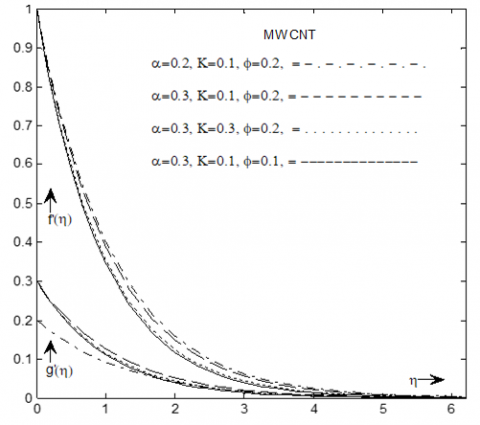Figure 3. Velocity for different parameters

The effect of local porosity parameter for SWCNT and MWCNT along axial and transverse direction leads to reduce the boundary layer thickness. Reason behind this is that an enhancement in CNTs enhances the boundary layer thickness. The influence of nanoparticle volume fraction for SWCNT and MWCNT along axial and transverse direction leads to increase the boundary layer thickness.

## figure_4.pngFigure 4. Velocity for different values of n

Figure 4 shows the influence of power law index on the velocity profile along axial and transverse direction for both carbon nanotubes. Velocity profile decreases when the power law index increases for both CNTs. From these results we found that velocity are higher for MWCNTs as compared with SWCNTs.

Figure 5 shows the influence of Biot number Bi on the temperature distribution.

It is noted that an enhance in Biot number causes enhancement in the temperature profile. Physically speaking, an enhance in Biot number decreases sheet’s thermal resistance and also an improves convective heat transfer to the fluid on the sheet. An increment in the Biot number relates to stronger convection which illustrates the higher temperature distribution and more thickness for both CNTs. The response of temperature profile for nanoparticle volume fraction is shown in Figure 6 This figure describes that, the temperature profile in the boundary layer is an increasing function of volume fraction parameter.

## figure_5.pngFigure 5. Temperature profile of Bi

## figure_6.png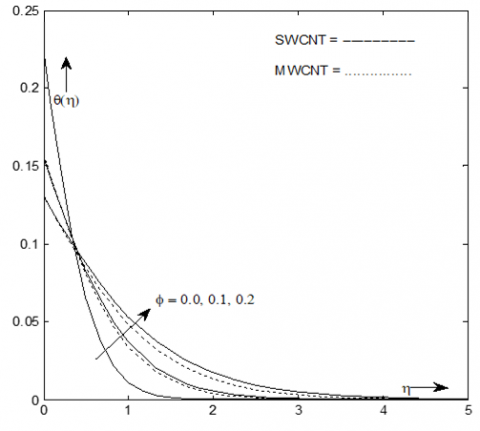Figure 6. Temperature profile of ϕ

## figure_7.png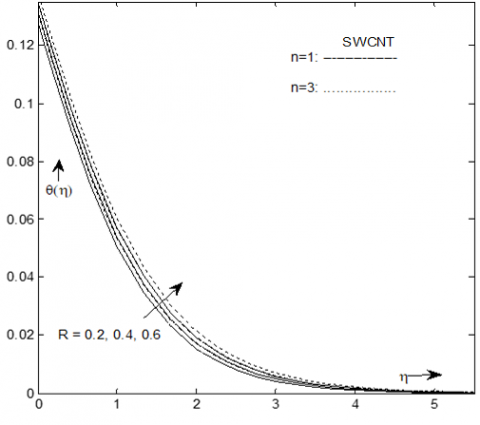Figure 7. Temperature profile of R  for SWCNT

## figure_8.pngFigure 8. Temperature profile of R  for MWCNT

## figure_9.png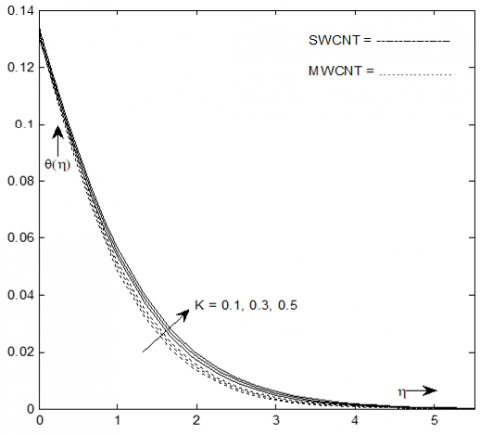Figure 9. Temperature profile of K

In Figure 9 both temperature profile and related layer thickness increase when the permeability parameter of porous media increases for both CNTs. Because an enhancement in carbon nanotubes increases the boundary layer thickness.

Figure 10 shows that the nanoparticle volume fraction parameter increases as entropy generation decreases. It is also noted that the entropy generation number reduces with the rising value of the nanoparticle volume fraction parameter due to the high dissipated energy resulted from the sharper velocity gradient near the wall. On the other hand, reverse effect has been obtained for far field. It is also noted that due to decrease in the friction between the stretching surface and the nanofluid results decrease in entropy production.

## figure_10.png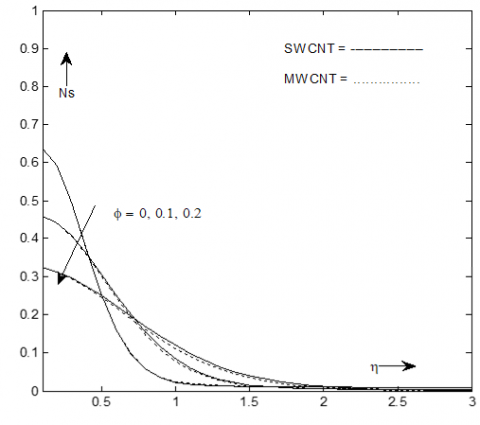Figure 10. Entropy for different values of ϕ

## figure_11.pngFigure 11. Entropy for different values of Bi

## figure_12.pngFigure 12. Entropy for different values of α

Figure 11 illustrates the effects of Biot number Bi on the entropy generation number. Near the stretching surface, the effects of Biot on entropy generation number are prominent. Increase in entropy generation number with an increase in the Biot number in the boundary layer region. In the region far away from the surface of the stretching sheet, the entropy generation is negligible. Therefore, the entropy can be minimized by increasing the convection through the boundary. Figure 12 displays that entropy generation decreases when ratio parameter increases.

## figure_13.png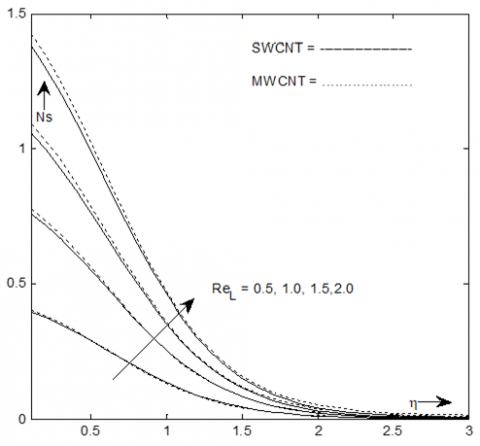Figure 13. Entropy for different values of ReL

## figure_14.pngFigure 14. Entropy for different values of $BrΩ^{-1}$

The impacts of the non-dimensional parameter Reynolds number and $BrΩ^{-1}$  on the entropy generation are presented in Figures 13 and 14 respectively. The rises in both non dimensional parameters resultant ris in the entropy generation. Figure 13 shows that Reynolds number increases due to higher heat transfer rate at the surface of stretching sheet. When the Reynolds number increases, the entropy due to the heat transfer becomes prominent, and fluid friction decrease near the stretching sheet. However when the distance increases from the surface of the stretching sheet, these effects are negligible. Reynolds number generates the higher entropy. Entropy function strongly depends upon Reynolds number. With high Reynolds number, hectic motion occurs because as Re increases, the fluid moves more disturbingly and thus contribution of fluid friction and heat transfer on entropy result tends to increase in entropy generation. Figure 14 shows that entropy generation increases when $BrΩ^{-1}$  increases, because of higher $BrΩ^{-1}$  increases the nanofluid friction. The relative significance of viscous consequence on the flow is determined by this parameter. In the figure, the entropy number is greater for high dimensionless group parameter. The fact that for higher dimensionless group parameter, the entropy number due to fluid friction is enhanced.

7. Conclusion

Entropy generation Analysis of water based Carbon Nanotubes nanofluid 3D flow over a inclined nonlinear stretching sheet embedded in porous media have been studied. The effects of different physical parameters on velocity, temperature and entropy profile have been examined. Following points has been concluded

• Velocity component reduces for higher stretching ratio parameter in the axial direction, while reverse effect has been shown in the transverse direction.
• Both velocity components are higher for MWCNTs as compared with SWCNTs.
• Temperature profile increases with increasing value of Biot number, porosity parameter and radiation parameter.
• The non-linear radiation has high effect on flow fields compared to linear thermal radiation.
• Entropy generation number increases with increasing value of Biot number, Reynolds number and $BrΩ^{-1}$ .
• The role of Reynolds number is to generate the higher entropy so entropy strongly depends on Reynolds number.

Entropy generation number reduces with increasing value of stretching ratio parameter and nanoparticle volume fraction.

Nomenclature
 K R α Pr ReL Br Ω Local porosity parameter Radiation parameter Ratio parameter Prandtl Number Reynolds number  Brinkmann number Dimensionless temperature difference Greek symbols θ Dimensionless temperature
References

 Choi SUS, Zhang ZG, Yu W, Lockwood FE, Grulke EA. (2001). Anomalous thermal conductivity enhancement in nanotube suspension. Applied Physics Lett 79(14): 2252-2254. http://dx.doi.org/10.1063/1.1408272

 Hayat T, Khan MI, Farooq M, Alsedi A, Yasmeen T. (2016). Impact of Margoni convection in the flow of carbon-water nanofluid with thermal radiation. Int. Journal of Heat and Mass Transfer 106: 810-815. https://doi.org/10.1016/j.ijheatmasstransfer.2016.08.115

 Aman S, Khan I, Ismail Z, Salleh MZ, Al-Mdallal QM. (2017). Heat transfer enhancement in free convection flow of CNTs Maxwell nanofluids with four different types of molecular liquids. Sci. Rep. 7(1): 2445. https://doi.org/10.1038/s41598-017-01358-3

 Hayat T, Ahmed S, Muhammad T, Alsedi A, Ayub M. (2017). Computational modelling for homogenous-heterogeneous reactions in three-dimensional flow of carbon nanotubes. Res. In Physics 7: 2651-2657. https://doi.org/10.1016/j.rinp.2017.07.040

 Jain S, Bohra S. (2018). Hall current and radiation effects on unsteady MHD squeezing nanofluid flow in a rotating channel with lower stretching permeable wall. Applications of Fluid Dynamics 127-141. http://dx.doi.org/10.1007/978-981-10-5329-0_9

 Hayat T, Aziz A, Muhammad T, Alsedi A. (2016). On magnetohydrodynamic three-dimensional flow of nanofluid over a convectively heated nonlinear stretching surface. Int. Journal of Heat and Mass Transfer 100: 566-572. http://dx.doi.org/10.1016/j.ijheatmasstransfer.2016.04.113

 Mustafa M, Mustaq A, Hayat T, Alsedi A. (2016). Rotating flow of magnetite-water nanofluid over a stretching surface inspired by non linear thermal radiation. PLOS ONE 11(2): e0149304. http://dx.doi.org/10.1371/journal.pone.0149304

 Gopal D, Kishan N, Raju CSK. (2017). Viscous and joule’s dissipation on Casson fluid over a chemically reacting stretching sheet with inclined magnetic field and multiple slips. Inform. in Med. Unlocked 9: 154-160. http://dx.doi.org/10.1016/j.imu.2017.08.003

 Kandasamy R, Vignesh V, Kumar A, Hasan SH, Isa NM. (2018). Thermal radiation energy due to SWCNTs on MHD nanofluid flow in the presence of seawater/water: Lie group transformation. Ain Shams Eng. Journal 9(4). http://dx.doi.org/10.1016/j.asej.2016.04.022

 Mahanthesh B, Gireesha BJ, Gorla RSR. (2016). Nonlinear radiative heat transfer in MHD 3-D flow of water based nanofluid over a non-linearly stretching sheet with convective boundary condition. Journal of Nige. Math. Soc 35: 178-198. http://dx.doi.org/10.1016/j.jnnms.2016.02.003

 Jain S, Choudhary R. (2015). Effects of MHD on boundary layer flow in porous medium due to exponentially shrinking sheet with slip. Journal of Procedia Eng 127: 1203-1210. http://dx.doi.org/10.1016/j.proeng.2015.11.464

 Chauhan DS, Olkha A. (2011). Slip flow and heat transfer of a second grade fluid in a porous medium over a stretching sheet with power law surface temperature or heat flux. J. of Chem. Eng. Communi 198(9): 1129-1145. https://doi.org/10.1080/00986445.2011.552034

 Nayak MK. (2017). MHD 3D flow and heat transfer analysis of nanofluid by shrinking surface inspired by thermal radiation and viscous dissipation. International Journal of Mech. Sciences 124-125: 185-193. http://dx.doi.org/10.1016/j.ijmecsci.2017.03.014

 Jain S, Bohra S. (2017). Heat and mass transfer over a three-dimensional inclined non-linear stretching sheet with convective boundary conditions. Indian Journal of Pure and Applied Physics 55: 847-856. http://op.niscair.res.in/index.php/IJPAP/article/view/15706/1411

 Matin MH, Nobari MRH, Jahangiri P. (2012). Entropy analysis in mixed convection MHD flow of nanofluid over a non-linear stretching sheet. Journal of Thermal Science and Technology 7(1): 104-119. http://dx.doi.org/10.1299/jtst.7.104

 Rehman AU, Mahmood R, Nadeem S. (2017). entropy analysis of radioactive rotating nanofluid with thermal slip. Applied Ther. Engineering 112: 832-840. http://dx.doi.org/10.1016/j.applthermaleng.2016.10.150

 Das S, Chakraborty S, Jana RN, Makinde OD. (2015). Entropy analysis of unsteady magneto-nanofluid flow past accelerating stretching sheet with convective boundary condition. Applied Math. Mech. Engl. Ed. 36(12): 1593-1610. http://dx.doi.org/10.1007/s10483-015-2003-6

 Chauhan DS, Kumar V. (2013). Entropy analysis for third grade fluid flow with temperature-dependent viscosity in annulus partially filled with porous medium. Theoret. Appl. Mech. 40(3): 441-464. http://dx.doi.org/10.2298/TAM1303441C

 Shirley A, Aurang Z. (2017). Entropy generation of nanofluid flow over a convectively heated stretching sheet with stagnation point flow having nimonic 80A nanoparticles: Buongiorno model. Fluid Mech. and Thermo 618-624.

 Vasanthakumari R, Pondy P. (2018). Mixed convection of silver and titanium dioxide nanofluids along inclined stretching sheet in presence of MHD with heat generation suction effect. Math. Modell. of Eng. Pro 5(2): 123-129. http://dx.doi.org/10.18280/mmep.050210